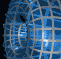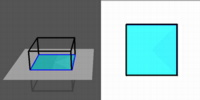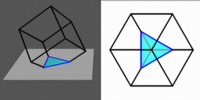Português | English Beyond 3D > Iced Cubes > Slicing 3D cube

# Slicing a Three-Dimensional CubeThis movie shows the cube passing through a plane face first. On the left is a view of the cube in perspective; on the right is a view from directly above which represents what a two-dimensional person viewing the cube from within the plane would be able to preceive. The portion of the cube that is above the plane is in black, while the part that is below is in grey. The slices are always squares, and so our two-dimensional person would see "a square existing for a while". In this case, time is being used at the third dimension.

` `This movie shows the cube passing through a plane edge first. Here, the slice begins as an edge, then becomes a rectangle; the rectangle grows, becomes a square for a moment, and then gets wider than it is tall. At its widest, it is as wide as the diagonal of one of the square faces of the cube. The rectangle then shrinks back to an edge at the top of the cube.

` `The most interesting slicing sequence for the cube is when it passes though a plane corner first. In this case, the initial contact is a point, which then becomes a small equilateral triangle. This triangle grows until it touches three of the corners of the cube (the three edges are sweeping out three of the faces of the cube, and are now half-way through each of these faces). At this point, the corners of the triangles begin to be cut off by the other three faces of the cube. The slice then becomes hexagonal, and at the half-way point, the slice is a regular hexagon, with the slice cutting each of the six faces of the cube in exactly the same way. As the cube progresses through the plane, the slice turns again into a cut-off triangle (but inverted with respect to the original one) and finally becomes an equilateral triangle once again as three more vertices pass through the plane. This triangle shrinks down to a point and disappears.

` `

See the description of movie formats and plug-ins
if you are having difficulty getting these movies to play.

```
```